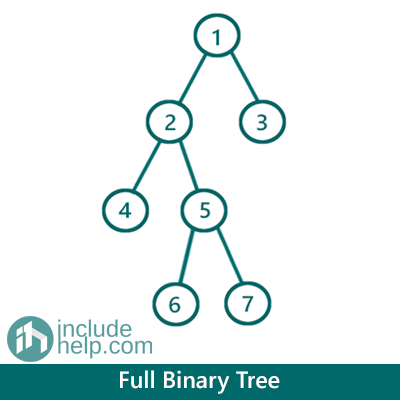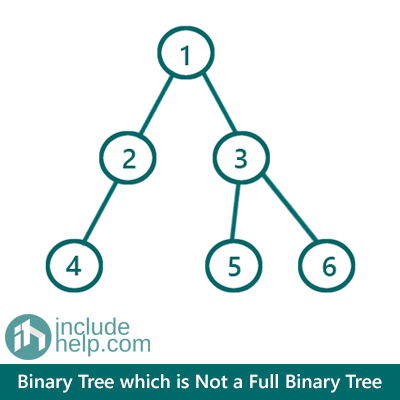# Full Binary Tree

In this article, we are going to see what Full Binary Tree is and how to check whether a binary tree is Full or not?
Submitted by Radib Kar, on July 31, 2020

Prerequisite: N-array Tree

## What is a Full Binary Tree?

A Full Binary Tree is a special kind of binary tree where each node has either 2 children or 0 children (leaf nodes).

For example, the below binary tree is a full binary tree whereas the second one is not.

Example 1:The above example is a Full Binary Tree as every node in this, either has two children or no children.

Example 2:The above binary tree is not a full binary tree as all its nodes don't have either 2 or 0 children. The node having value 2 violates the condition as it has single child only (4). Don't think NULL is a child!

## Properties for Full binary tree

There are few properties for Full binary tree which have been listed below:

Say,

Number of Total Nodes = N
Number of internal Nodes= I
Number of leaf Nodes= L

1. In Full binary tree each internal node(which are not leaf nodes) has exact 2 child
2. Number of Leaf nodes= Number of internal nodes +1 or L=I+1
3. Number of Internal Nodes= (Number of Total Nodes-1)/2 or I=(N-1)/2
4. Number of Leaf nodes = (Number of Total Nodes+1)/2 or L=(N+1)/

## Check whether a Binary Tree is Full binary tree or not

1) Recursive implementation:

Comments are there to show how it's working.

```#include <bits/stdc++.h>
using namespace std;

class TreeNode {
public:
int val;
TreeNode* left;
TreeNode* right;

TreeNode(int v)
{
val = v;
left = NULL;
right = NULL;
}
};

//Full Binary Tree checking recursively
bool is_Full_Binary_Tree(TreeNode* root)
{
if (!root)
return true;

//if its a leaf node then fine, return true
if (!root->left && !root->right) {
return true;
}

/*
if it has only one children then return False
since in the previous step, we had checked for that "&&"" condition
it's guaranteed that both is Not NULL if control reaches here
so "||" will actually check whether anyone is NULL and other one not
if both is not not NULL it's doesn't enter the loop
*/
if (!root->left || !root->right) {
return false;
}

/*
if control reaches here that means the current node
has two children( Not NULL), so now check whether
the subtrees are FULL or NOT, both of the subtrees
need to be Full binary tree themselves and
we can check that recursively
*/
return is_Full_Binary_Tree(root->left) && is_Full_Binary_Tree(root->right);
}

int main()
{
//Example 1 Tree is built
TreeNode* root1 = new TreeNode(1);

root1->left = new TreeNode(2);
root1->right = new TreeNode(3);
root1->left->left = new TreeNode(4);
root1->left->right = new TreeNode(5);
root1->left->right->left = new TreeNode(6);
root1->left->right->right = new TreeNode(7);

if (is_Full_Binary_Tree(root1))
cout << "The tree is Example 1 is a Full Binary Tree\n";
else
cout << "The tree is Example 1 is not a Full Binary Tree\n";

//Example 2 Tree is built
TreeNode* root2 = new TreeNode(1);

root2->left = new TreeNode(2);
root2->right = new TreeNode(3);
root2->left->left = new TreeNode(4);
root2->right->left = new TreeNode(5);
root2->right->right = new TreeNode(6);

if (is_Full_Binary_Tree(root2))
cout << "The tree in Example 2 is a Full Binary Tree\n";
else
cout << "The tree in Example 2 is not a Full Binary Tree\n";

return 0;
}
```

Output:

```The tree is Example 1 is a Full Binary Tree
The tree in Example 2 is not a Full Binary Tree
```

2) Using iterative Approach

Comments are there to show how it's working.

```#include <bits/stdc++.h>
using namespace std;

class TreeNode {
public:
int val;
TreeNode* left;
TreeNode* right;

TreeNode(int v)
{
val = v;
left = NULL;
right = NULL;
}
};

//Full Binary Tree checking iteratively
bool is_Full_Binary_Tree(TreeNode* root)
{
if (!root)
return true;

queue<TreeNode*> q;
q.push(root);
while (!q.empty()) {
TreeNode* temp = q.front();
q.pop();
//if leaf node
if (!temp->left && !temp->right)
continue;
//if current node has only one child and another is NULL
else if (!temp->left || !temp->right)
return false;
else { //it has both the left & right child, so push both
q.push(temp->left);
q.push(temp->right);
}
}

return true;
}

int main()
{
//Example 1 Tree is built
TreeNode* root1 = new TreeNode(1);
root1->left = new TreeNode(2);
root1->right = new TreeNode(3);
root1->left->left = new TreeNode(4);
root1->left->right = new TreeNode(5);
root1->left->right->left = new TreeNode(6);
root1->left->right->right = new TreeNode(7);

if (is_Full_Binary_Tree(root1))
cout << "The tree is Example 1 is a Full Binary Tree\n";
else
cout << "The tree is Example 1 is not a Full Binary Tree\n";

//Example 2 Tree is built
TreeNode* root2 = new TreeNode(1);
root2->left = new TreeNode(2);
root2->right = new TreeNode(3);
root2->left->left = new TreeNode(4);
root2->right->left = new TreeNode(5);
root2->right->right = new TreeNode(6);

if (is_Full_Binary_Tree(root2))
cout << "The tree in Example 2 is a Full Binary Tree\n";
else
cout << "The tree in Example 2 is not a Full Binary Tree\n";

return 0;
}
```

Output:

```The tree is Example 1 is a Full Binary Tree
The tree in Example 2 is not a Full Binary Tree
```